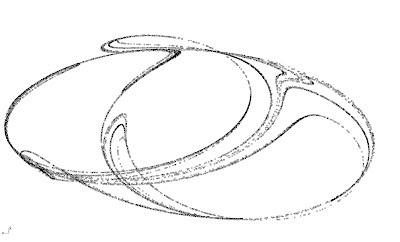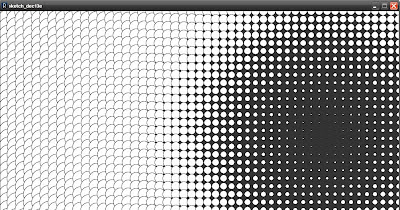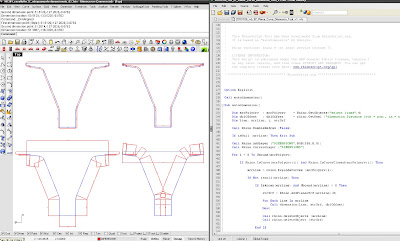## 14 December 2010

### Peter de Joung attractor 02//////////////////////////////////Peter de Joung attractor scripted in Processing and imported in Gh via UDP receiver.
A cloud of 3D points is created via an Autobake VB custom script.

----------------------------------------------------------------------------------------------------

Author: davide del giudice

web: http://www.co-de-it.com/
----------------------------------------------------------------------------------------------------
www.livearchitecture.net

----------------------------------------------------------------------------------------------------
Autobake VB script
modified and simplify from an origianl code of http://www.thediscontinuum.net/

----------------------------------------------------------------------------------------------------
--------------------------------------------------------------------------------------------------------------------------------------------------------------------------------------------------------
Grasshopper 3dPoint Autobake Code: ----------------------------------------------------------------------------------------------------
Private Sub RunScript(ByVal x As List(Of On3dPoint), ByRef A As Object)
Dim point As Object
For j As Integer = 0 To x.count - 1
Next
A = Nothing End Sub## 13 December 2010

### Peter de Joung attractor 01//////////////////////////////////From this old post on Peter de joung attractor in Rhinoscript I started to work on a code in Processing, in order to create a simulation of this matemathical attractor with a huge amount of particles.Different effects changing variables.

Here the script:

//code by Davide del giudice
//Peter de joung attractor_101015

float x,y,xn,yn;
float a,b,c,d;
color bla;
float i=0;

void setup()
{
size (1000,1000);
//smooth();
frameRate(60);
bla= color(0,150,255);
//background(0);
x=0;
y=10;
a=1.4;
b=1.56;
c=1.4;
d=-6.56;

}
void draw()
{

background(0);
//stroke(0,90);
fill(175,40);
//c=3*cos(i);
//d=3*sin(i);
//a=3*cos(i);

for(float i=0;i<10000;i++)
{ xn=x; yn=y;
x=(d*sin(a*xn)-sin(b*yn));
y=(c*cos(a*xn)+cos(b*yn));
fill(0,150,255);
//ellipse(width/2+200*x,height*0.7+200*y,5,5);
set(int(width/2+200*x*mouseX/1000),int(height/1.5+200*y),bla);
i++;
}
}

### Processing_getting started

Some Processing exercises done in September when I started to approach to this software.
They are very simple sketches, just for fun and to understand the grammar of javascript code. I'd to push forward my research with this software working trough some recursive script, agents and metaball. TBC...Follow the circle //
A circle follows the mouse pointer and changes it size following the sin math formula. At the right side of the screen the circle draws a traces of the movement.Bounce turbo //
A circle bounces fast into the canvas.Matrix change color //
A matrix of circles that change colors following the mouse pointer.Strange ellipse //
Curious effects from a code that draws a trace of the ellipse moved by mouse pointer. The ellipse changes color from white to black if mouse is pressed.Continuous mode//
An ellipse moving from x=0 to x= widt canvas in a continous mode scaling itself trough a sin math formula.Spot class//
Generation of rectangles that change position, dimension and color.Point attractor//
A simulation of a point attractor into a matrix of circles.

Some codes posted are modified from original codes of Supermanouvre blog or from the official site Proccesing.org.

### RH AutoDimension 02//////////////////////////////////////////

A new improvement from my AutoDimension rhinoscript. Thanks to Jan Hempel, whom wrote this script, you can choose the distance of the dimension from the line, height of text and if your dimension is in or out a closed planar shape.